### SPC's

The first one is the cataloguing algorithm for SPC's (single point constraints, *BOUNDARY). Let's say a boundary condition m is defined for node i in direction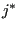. According to the input deck rulescan take the following values:

• For structures:
• 0 or 11: temperature
• 1..3: translational dofs
• 4..6: rotational dofs
• For networks:
• 0 or 11: total temperature
• 1: mass flow
• 2: total pressure
• For 3-dimensional CFD-calculations:
• 0 or 11: static temperature
• 1..3: velocity
• 8: static pressure
• For electromagnetic calculations:
• 8: electric potential

The valueis first mapped onto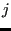using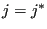except for:

•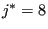is mapped onto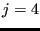•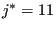is mapped onto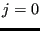(11 was kept out of compatibility with Abaqus).

Since static pressure is only used for fluids, rotations only for structures, and the electric potential only for electromagnetic calculations the triple use of dof 4 is no problem: it is not possible that a pressure and a rotation is applied in the same node. The same applies to the other degrees of freedom. For instance,j=2 can be a displacement (in global y-direction) in a structural node, a total pressure in a network node or a velocity (in global y-direction) in a CFD-calculation.

Then, a degree of freedom idof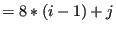is assigned to this boundary condition. Subsequently, it is stored at location k in the one-dimensional field ikboun, where all previous boundary degrees of freedom are stored in numerical order such that ikboun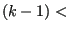idof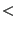ikboun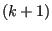. Furthermore the number of the boundary condition (m) is stored in ilboun: ilboun(k)=m, and the node of the boundary condition, its direction and value are stored in the one-dimensional fields nodeboun, ndirboun and xboun: nodeboun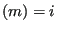, ndirboun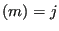and xboun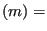value. If an amplitude definition applies to the boundary condition, its number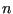is stored in the one-dimensional field iamboun: iamboun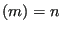.

The SPC type is stored in the one-dimensional field typeboun. SPC's can be of different types, depending on whether the were defined by a genuin *BOUNDARY CARD, or introduced for other reasons. The field typeboun is a one-dimensional character*1 field. Other reasons to introduce SPC's are:

• fixing of the midplane in expanded plane stress/plane strain/axisymmetric elements
• taking care of the inhomogeneous term in nonlinear MPC's such as the PLANE *MPC, the STRAIGHT *MPC, a *RIGID BODY definition or USER *MPC.

The corresponding type code is:

• B = prescribed boundary condition
• M = midplane constraint (plane stress/plane strain/axisymmetric elements)
• P = PLANE MPC
• R = RIGID BODY definition
• S = STRAIGHT MPC
• U = USER MPC

The total number of boundary conditions is stored in variable nboun.

Consequently, ikboun contains all degrees of freedom of the boundary conditions in numerical order, and ilboun contains the corresponding boundary condition numbers. This assures that one can quickly check whether a given degree of freedom was used in a SPC. For example, if the SPC's look like:

*BOUNDARY
8,1,1,0.
10,1,2,0.
7,3,3,-1.


the fields look like:

 nodeboun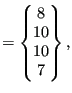ndirboun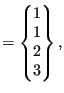xboun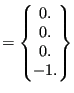(663)

 typeboun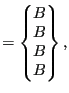ikboun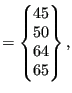ilboun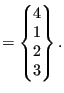(664)

and nboun=4.

Finally, the following one-dimensional fields are also used:

• nodebounold: contains the node numbers of the SPC's at the end of the last step
• ndirbounold: contains the directions of the SPC's at the end of the last step
• xbounold: contains the values of the SPC's at the end of the last step, or, if this is the first step, zero values.
• xbounact: contains the values of the SPC's at the end of the present increment, or, for linear calculations, at the end of the present step. The field xbounact is derived from the fields xbounold and xboun by use of the present time and/or amplitude information. How this is done depends on the procedure and is explained later on.
• xbounini: contains the values of the SPC's at the end of the last increment, or, if this is the first increment in the first step, zero's. This field is used for nonlinear calculations only.

Notice that among the boundary conditions SPC's are somewhat special. They are sometimes called geometric boundary conditions to distinguish them from the natural boundary conditions such as the application of a concentrated or distributed load. To remove a natural boundary condition, just set it to zero. This is not true for geometric boundary conditions: by setting a SPC to zero, the corresponding node is fixed in space which usually does not correspond to what one understands by removing the SPC, i.e. free unconstrained motion of the node. Therefore, to remove a SPC the option OP=NEW must be specified on the *BOUNDARY keyword card. This removes ALL SPC constraints. Then, the constraints which the user does not wish to remove must be redefined. Depending on the procedure (*STATIC, *DYNAMIC...), the change of SPC's is applied in a linear way. This means that the old SPC information must be kept to establish this linear change. That's why the fields nodebounold and ndirbounold are introduced. The relationship between the old and new SPC's is established in subroutine spcmatch, called from ccx_2.19.c.